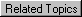# Limitations on CGI scripts

The following limitations will be placed on ALL CGI scripts:

MAXIMUM NUMBER OF PROCESSES = 32 Processes

This is the number of processes that can be created by the CGI program at a given time.

CPU TIME = 10 Seconds

This is the amount of CPU time one CGI program can use at a given time.

MAXIMUM FILE SIZE = 100 KBytes

This is the maximum size that one CGI script can reach.

MAXIMUM STACK SIZE = 8 MBytes

This is the amount of stack memory that can be used by any CGI program.

MAXIMUM NUMBER OF OPEN FILES = 32 Open Files

This is the number of files that can be opened by the CGI program at a given time.